2022: SklogWiki celebrates 15 years on-line

# Kern and Frenkel patchy model

The Kern and Frenkel  patchy model is an amalgamation of the hard sphere model with attractive square well patches (HSSW). The potential has an angular aspect, given by (Eq. 1)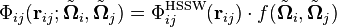$\Phi_{ij}({\mathbf r}_{ij}; \tilde{ {\mathbf \Omega}}_i, \tilde{ {\mathbf \Omega}}_j) =\Phi_{ij}^{ \mathrm{HSSW}}({\mathbf r}_{ij}) \cdot f(\tilde{ {\mathbf \Omega}}_i, \tilde{ {\mathbf \Omega}}_j)$

where the radial component is given by the square well model (Eq. 2)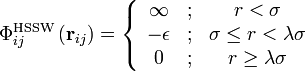$\Phi_{ij}^{ \mathrm{HSSW}} \left({\mathbf r}_{ij} \right) = \left\{ \begin{array}{ccc} \infty & ; & r < \sigma \\ - \epsilon & ; &\sigma \le r < \lambda \sigma \\ 0 & ; & r \ge \lambda \sigma \end{array} \right.$

and the orientational component is given by (Eq. 3)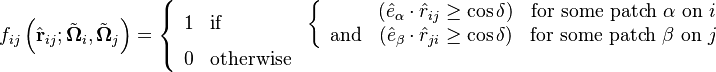$f_{ij} \left(\hat{ {\mathbf r}}_{ij}; \tilde{ {\mathbf \Omega}}_i, \tilde{ {\mathbf \Omega}}_j \right) = \left\{ \begin{array}{clc} 1 & \mathrm{if} & \left\{ \begin{array}{ccc} & (\hat{e}_\alpha\cdot\hat{r}_{ij} \geq \cos \delta) & \mathrm{for~some~patch~\alpha~on~}i \\ \mathrm{and} & (\hat{e}_\beta\cdot\hat{r}_{ji} \geq \cos \delta) & \mathrm{for~some~patch~\beta~on~}j \end{array} \right. \\ 0 & \mathrm{otherwise} & \end{array} \right.$

where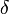$\delta$ is the solid angle of a patch (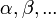$\alpha, \beta, ...$) whose axis is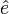$\hat{e}$ (see Fig. 1 of Ref. 1), forming a conical segment.

## Two patches

The "two-patch" Kern and Frenkel model has been extensively studied by Sciortino and co-workers .

## Four patches

Main article: Anisotropic particles with tetrahedral symmetry

## Single-bond-per-patch-condition

If the two parameters$\delta$ and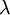$\lambda$ fullfil the condition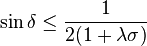$\sin{\delta} \leq \dfrac{1}{2(1+\lambda\sigma)}$

then the patch cannot be involved in more than one bond. Enforcing this condition makes it possible to compare the simulations results with Wertheim theory 

## Hard ellipsoid model

The hard ellipsoid model has also been used as the 'nucleus' of the Kern and Frenkel patchy model .